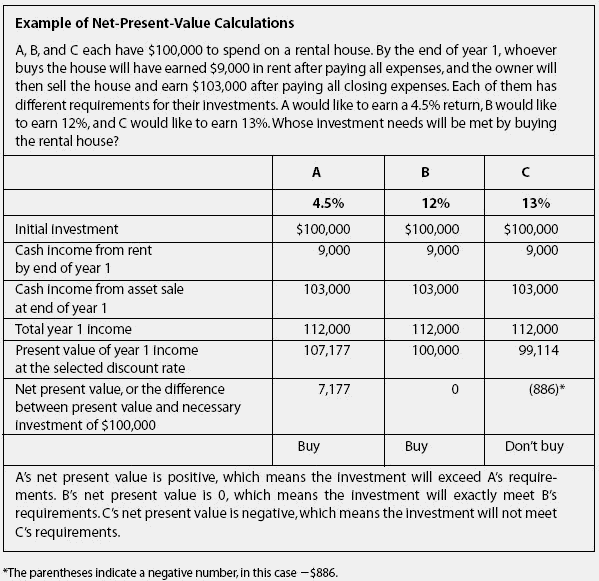# net present value

Also found in: Dictionary, Acronyms, Encyclopedia, Wikipedia.

## Net present value (NPV)

The present value of the expected future cash flows minus the cost.

## Net Present Value

A measure of discounted cash inflow to present cash outflow to determine whether a prospective investment will be profitable. For example, if a dentist wishes to purchase a new dental practice, he may calculate the net present value over a number of years to see if he will recover his investment in a reasonable period of time. If the ask price for the dental practice is \$500,000, this is the present cash outflow used in the calculation. If the discounted cash inflow over, say, two years, is greater than or equal to \$500,000, then the investment will likely be profitable. See also: Present value.

## net present value

The discounted value of an investment's cash inflows minus the discounted value of its cash outflows. To be adequately profitable, an investment should have a net present value greater than zero. For investment in securities, the initial cost is usually the only outflow.

## net present value

see INVESTMENT APPRAISAL, DISCOUNTED CASH FLOW.

## net present value

see DISCOUNTED CASH FLOW.

## net present valueAn analytical tool for evaluating whether or not to purchase an investment. The tool does not tell you if an investment is good or bad;it tells you if the investment will meet your predetermined objectives or not.

• Critical to defining your objectives is setting the equivalent of an interest rate you would like to earn on your initial cash investment. This is called the discount rate. The discount rate may change from investment to investment, depending on your assessment of the risk. The safest investment is an FDIC-insured savings account, but it returns the lowest interest rate. You, the investor, decide what rate you would like to earn. You use the net-present-value tool to calculate whether a particular investment will earn the rate you want.

• Having said all that, the official definition of net present value is as follows: using a preselected discount rate, net present value is the present value of all cash incomes, less the present value of all cash outflows (including initial investment). If this is not clear, it will become so with the example below.

• If the net present value is 0 or a positive number, the investor should go forward. If the answer is negative for the discount rate selected, then the investment should not be made because it will not meet the investor's objectives, not because it is a “bad investment” in the ordinary sense of that phrase.

• The Excel formula for present value is pv (rate, cashflow, cashflow, cashflow)
‘'Rate” is the cell with the interest rate the investor would like to earn. Each of the “cashflow” entries is a cell address for cash flows by the end of each year, such as year 1, year 2, year 3, and so on. If an asset is sold in a particular year, the net sales price (after expenses of the sale) is entered as part of the cash flow for that year. One flaw of the system is that it assumes all cash flows are received at year-end, when they are really received over time, but that is usually a relatively minor problem.

References in periodicals archive ?
Then, I calculate the interest rate that would be required to make the Net Present Value of each project zero.
In order to conduct a net present value analysis, the costs of constructing and maintaining the streambank stabilization area must be calculated.
The equation for the net present value is similar to the IRR equation:
Figure 2: Calculating the Net Present Value of the Investment
Instead of simply adding together each year's cost or benefit, net present value analysis first converts the value of future costs and benefits to their actual value today.
We will discuss three different scenarios of fiscal concession to compute the net present value of a project in the developed and underdeveloped area.
The net present value approach discounts the expected cash flows from each year of the project.
Table 1 Example of net present value calculation (\$000) Year 0 1 2 3 4 Capital (400) 0 0 0 0 outlay Revenue 500 500 500 500 500 Operating (200) (200) (200) (200) (200) expenses Cash flow (100) 300 300 300 300 Present value (100) 263 231 202 178 @ 14%.
For fruit and rubber, the net present value is \$6,330 per hectare.
a net present value can be determined by subtracting the discounted (or present value) costs from the discounted (or present value) benefits.

Site: Follow: Share:
Open / Close